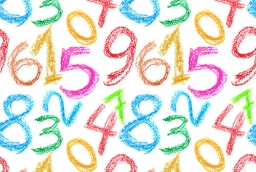# Consecutive 21043

The sum of three consecutive natural numbers is 864. Determine the smallest of them.

x =  287

### Step-by-step explanation:

x+(x+1)+(x+2)=864

3x = 861

x = 861/3 = 287

x = 287

Our simple equation calculator calculates it.Did you find an error or inaccuracy? Feel free to write us. Thank you!

Tips for related online calculators
Do you have a linear equation or system of equations and looking for its solution? Or do you have a quadratic equation?